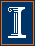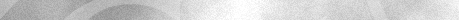Econ 508 Econometrics Group Home | Faculty | Students | Alumni | Courses | Research | Reproducibility | Lab | Seminars | Economics | Statistics | Fame
Applied Econometrics
Econ 508 - Fall 2007

e-Tutorial 8: Granger CausalityWelcome to the eight issue of e-Tutorial. This issue focuses on time series models, with special emphasis on the tests of Granger causality. I am providing instructions for both R and STATA. I would like to remark that the theoretical background given in class is essential to proceed with the computational exercise below. Thus, I recommend you to study Prof. Koenker's Lectures Note 9 as you go through the tutorial.

The first thing you need is to download the data in text format by clicking here., or from the Econ 508 web site (Data).  Save it in your preferred directory (I will save my as "C:/eggs.txt".)

I suggest you to open the file in Notepad (or another text editor) and type the name of the variable "year" in the first row, first column, before "chic" "egg". Use <Tab> to separate the names of variables. Save the file (I will save mine as C:/eggs1.txt).

Inserting the Data in R

Next, you need to import that data set to R, using the following commands:
year<-Thurman\$year
egg<-Thurman\$egg
chic<-Thurman\$chic

It is useful to call the time series package, and declare chickens and eggs as time series:
library(ts)
year<-ts(year)
chic<-ts(chic)
egg<-ts(egg)

Inserting the Data in Stata:

In Stata, you can type:
infile year chic egg using"C:/eggs1.txt"

You will see that, because I included variables names in the first row of the file egg1.txt, Stata reads the first line of the data set as missing values. You should delete this line (only!) on the Stata data editor window. Next you need to declare your data as time series:
tsset year

I. Granger Causulatity

In the problem set 3 you will be asked to replicate the results of Thurman and Fisher's (1988), Table 1. I recommend you to sketch the Granger test, explain the NULL and the ALTERNATIVE hypotheses, and run the test for the causality for all lags, and both directions. At each round, collect the F-test statistics, p-values, and R-squares. At the end, please provide a table in the same format of Thurman and Fisher's (1988), containing your results, along with a graphical analysis.

You have the option to run the Granger causality tests in in either R or Stata.

In R: There is a code for the Granger test as follows:

#Copy from this point:
"granger" <-function(d, L, k = 1)
{
#d is a bivariate time-series:  regress d[,k] on L lags of d[,1] and d[,2].
#This is a modified version for R, in which the command ts.matrix was substituted by ts.intersect.
names.d <- dimnames(d)[]
D <- d
for(i in 1:L)
{
D <-ts.intersect(D, lag(d,  - i))
#don't you find -1 bizarre here and it annoying to need the loop
}
dimnames(D)[] <- paste(rep(names.d, L + 1), "_", rep(0:L, times = rep(2, L + 1)), sep = "")
y  <- D[, k]
n  <- length(y)
x1 <- D[,  - (1:2)]
x0 <- x1[, ((1:L) * 2) - (k %% 2)]
z1 <- lm(y ~ x1)
z0 <- lm(y ~ x0)
S1 <- sum(z1\$resid^2)
S0 <- sum(z0\$resid^2)
ftest <- ((S0 - S1)/L)/(S1/(n - 2 * L - 1))
list(ftest = ftest, p.val = 1 - pf(ftest, L, n - 2 * L - 1), R2 = summary(z1)\$r.squared)
}
#To this point.

This code is available at http://www.econ.uiuc.edu/~econ472/routines.html., under the name granger.R. Your job is to copy the routine above and past it in the R console. (It's better to copy granger.R from the routines web page, because there the lines are not discontinuous...). This will create a function called "granger" that does the test for you. Next you should start running the Granger causality test for each of the lags and directions.

For example, to test if chickens Granger cause eggs, using 1 lag, you type:

granger(cbind(egg,chic), L=1)
\$ftest
 0.04703186

\$p.val
 0.8291935

\$R2
 0.9626998

and obsviously, to test in the reverse direction, you type:

granger(cbind(chic, egg), L=1)
\$ftest
 1.2071

\$p.val
 0.2771696

\$R2
 0.7250355

Do that for the for lags 1,2,3, and 4, and provide a table like Thurman and Fisher's (1988), containing your results, plus a graphical analysis.

In STATA: The first thing to do is to use the command summarize, detail or other functions presented in the previous tutorials, to obtain a description of the data. Once again, it is required  that you show explicitly what are the NULL and ALTERNATIVE hypotheses of this test, and the regression equations you are going to run. The results of Thurman and Fisher's (1988), Table 1, can be easily replicated using OLS regressions and the time series commands introduced in the previous tutorials.

A simple example in Stata:

*Causality direction A: Do chickens Granger-cause eggs? For example, using the number of lags equals 1 you proceed as follows:

regress  egg L.egg L.chic

Source |       SS       df       MS                  Number of obs =      53
---------+------------------------------               F(  2,    50) =  645.24
Model |  38021977.8     2  19010988.9               Prob > F      =  0.0000
Residual |  1473179.16    50  29463.5832               R-squared     =  0.9627
Total |  39495157.0    52   759522.25               Root MSE      =  171.65

------------------------------------------------------------------------------
egg      |      Coef.   Std. Err.       t     P>|t|       [95% Conf. Interval]
---------+--------------------------------------------------------------------
egg      |
L1 |   .9613121    .027241     35.289   0.000        .906597    1.016027
chic     |
L1 |  -.0001136   .0005237     -0.217   0.829      -.0011655    .0009383
_cons    |   279.3413   279.6937      0.999   0.323        -282.44    841.1226
------------------------------------------------------------------------------

And you can test if chickens Granger cause eggs using a F-test:

test L.chic

( 1)  L.chic = 0.0

F(  1,    50) =    0.05
Prob > F =    0.8292

**Causality direction B: Do eggs Granger-cause chickens? This involves the same techniques, but here you need to regress chickens against the lags of chickens and the lags of eggs. For example, using one lag you have:

regress  chic L.egg L.chic

Source |       SS       df       MS                  Number of obs =      53
---------+------------------------------               F(  2,    50) =   65.92
Model |  8.0984e+10     2  4.0492e+10               Prob > F      =  0.0000
Residual |  3.0712e+10    50   614248751               R-squared     =  0.7250
Total |  1.1170e+11    52  2.1480e+09               Root MSE      =   24784

------------------------------------------------------------------------------
chic     |      Coef.   Std. Err.       t     P>|t|       [95% Conf. Interval]
---------+--------------------------------------------------------------------
egg      |
L1 |   -4.32139   3.933252     -1.099   0.277      -12.22156     3.57878
chic     |
L1 |   .8349305    .075617     11.042   0.000       .6830493    .9868117
_cons    |   88951.72   40384.25      2.203   0.032       7837.569    170065.9
------------------------------------------------------------------------------

test L.egg

( 1)  L.egg = 0.0

F(  1,    50) =    1.21
Prob > F =    0.2772

Are those numbers similar to those you have obtained using R?

Do that for the for lags 1,2,3, and 4. Please provide a table in the same format of Thurman and Fisher's (1988), containing your results, plus a graphical analysis.

Causality in further lags: To test Granger causality in further lags, the procedures are the same. Just remember to test the joint hypothesis of non-significance of the "causality" terms.

Example: Do eggs Granger cause chickens (in four lags)?

regress  chic L.egg L2.egg L3.egg L4.egg L.chic L2.chic L3.chic L4.chic

Source |       SS       df       MS                  Number of obs =      50
---------+------------------------------               F(  8,    41) =   22.75
Model |  8.9451e+10     8  1.1181e+10               Prob > F      =  0.0000
Residual |  2.0154e+10    41   491569158               R-squared     =  0.8161
Total |  1.0961e+11    49  2.2369e+09               Root MSE      =   22171

------------------------------------------------------------------------------
chic     |      Coef.   Std. Err.       t     P>|t|       [95% Conf. Interval]
---------+--------------------------------------------------------------------
egg      |
L1 |   87.38472   26.87471      3.252   0.002       33.11014    141.6593
L2 |  -62.49408   41.76817     -1.496   0.142      -146.8466    21.85845
L3 |  -8.214513   44.09684     -0.186   0.853      -97.26989    80.84086
L4 |  -22.63552   30.59828     -0.740   0.464         -84.43    39.15897
chic     |
L1 |   .2332566   .1934323      1.206   0.235      -.1573878     .623901
L2 |     .45797   .2039095      2.246   0.030       .0461663    .8697736
L3 |  -.0184877   .2059394     -0.090   0.929      -.4343907    .3974153
L4 |   .0256691   .1779262      0.144   0.886      -.3336602    .3849984
_cons    |   147330.3   46385.32      3.176   0.003        53653.2    241007.3
------------------------------------------------------------------------------

and then test the joint significance of all lags of eggs

test L.egg L2.egg L3.egg L4.egg

( 1)  L.egg = 0.0
( 2)  L2.egg = 0.0
( 3)  L3.egg = 0.0
( 4)  L4.egg = 0.0

F(  4,    41) =    4.26
Prob > F =    0.0057

The same result could be obtained in R:

granger(cbind(chic, egg), L=4)
\$ftest
 4.256766

\$p.val
 0.005671437

\$R2
 0.8161197Last update: September 25, 2007